# C Program to Copy One String to Another

In this tutorial, we will learn about how to copy one string to another in the C language, with and without using the library function strcpy(). At last, we will also learn how to copy strings using pointers. Let's first start with a copy string using the library function.

## In C, use the strcpy() function to copy a string

To copy a string in C programming, you have to ask the user to enter the string to store it in the first string variable, say str1, and then copy it into the second string variable, say str2, using the strcpy() function of the string.h library. The function strcpy() takes two arguments. The value of the second argument gets copied to the first argument.

```#include<stdio.h>
#include<conio.h>
#include<string.h>
int main()
{
char str1, str2;
printf("Enter the string: ");
gets(str1);
printf("\nString 1 = %s", str1);
strcpy(str2, str1);
printf("\nString 2 = %s", str2);
getch();
return 0;
}```

As the above program was written in the Code::Blocks IDE, here is the first screenshot of the sample run that you will also see on your output screen after running the program: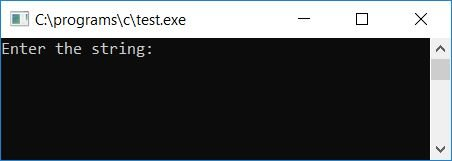Now enter any string, such as codescracker, and press the ENTER key to see the output shown here.This is the second snapshot of the sample run: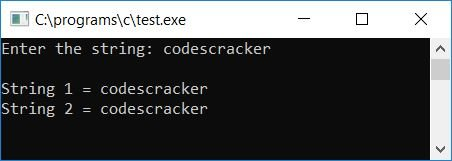Here is another sample run, where the user enters any string that contains space: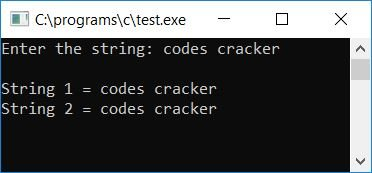#### Program Explained

1. Get string input from the user using the gets() function.
2. Before using the gets() function, include a string.h library function.
3. Now copy the string into another variable, say str2, using the strcpy() function.
4. The strcpy() function takes two arguments. The first argument is the target variable where the string is going to be copied.
5. The second argument is the source variable. The value of this variable is initialized to the target variable.
6. And finally, print the value of both variables.

As you can see from the previous program, I used the gets() function to get the string input. Because if we use the scanf() function and the user enters a string that contains spaces, then after the space all the string's values get skipped. For example, if the above program is replaced with the following program::

```#include<stdio.h>
#include<conio.h>
int main()
{
char str1, str2;
printf("Enter the string: ");
scanf("%s", str1);
printf("\nString 1 = %s", str1);
strcpy(str2, str1);
printf("\nString 2 = %s", str2);
getch();
return 0;
}```

Now let's take a sample run of the above program. In this case, I've provided the input as codes cracker. The string contains spaces; therefore, after the spaces, the rest of the string value gets skipped and only "codes" are initialized to the variable str1, as shown in the following snapshot:As you can see from the above snapshot, only "codes" are initialized in the variable str1, and the value of str1 gets copied to the str2 variable. Therefore, the above program is correct only when the user doesn't supply any strings with spaces.

## Copy a string in C without using the strcpy() function

This program performs the same function as the previous one, but without the use of the library function strcpy():

```#include<stdio.h>
#include<conio.h>
int main()
{
char strOrig, strCopy, i;
printf("Enter any string: ");
gets(strOrig);
for(i=0; strOrig[i]!='\0'; i++)
strCopy[i] = strOrig[i];
strCopy[i] = '\0';   // do not forget to set a null-terminated character at the end of string
printf("\nString 1 = %s", strOrig);
printf("\nString 2 = %s", strCopy);
getch();
return 0;
}```

Here is the final snapshot of the first sample run: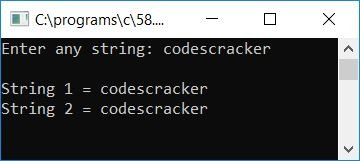Here is the final snapshot of the second sample run: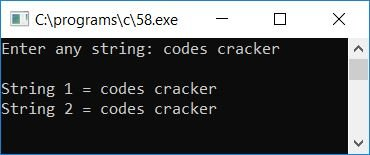#### Program Explained

• Get a string value using the gets() function.
• Start the for loop at the first character of the given string, strOrig, and continue until the null terminating character ('\0') occurs.
• Inside the for loop, copy each and every character of the given string, say strOrig, to any string, say strCopy, one by one.
• After exiting the for loop, insert a null-terminated character ('\0') at the end of the string (strCopy), into which all the characters of the given string (strOrig) are copied.
• Now print the value of both strings.

## Using a Pointer to Copy a String in C

Now, let's make the same program with a pointer:

```#include<stdio.h>
#include<conio.h>
void copystr(char *, char *);
int main()
{
char sourceStr, targetStr;
printf("Enter the string: ");
gets(sourceStr);
printf("\nString 1 = %s", sourceStr);
copystr(targetStr, sourceStr);
printf("\nString 2 = %s", targetStr);
getch();
return 0;
}
void copystr(char *targetString, char *sourceString)
{
while(*sourceString)
{
*targetString = *sourceString;
sourceString++;
targetString++;
}
*targetString = '\0';
}```

Here is the sample run: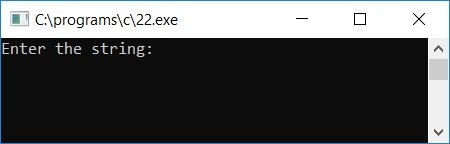Now enter any string, say "codescracker," and press the ENTER key to see the output as given in the second screenshot here: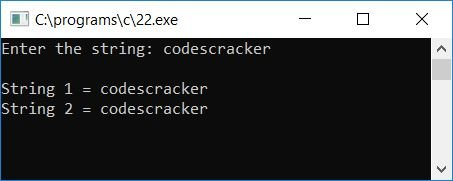#### Program Explained

1. Here we've created a function named copystr() that has two pointer arguments.
2. The first argument is responsible for the target string, and the second one is for the source string.
3. After calling the function copystr(), the target string and source string get passed as arguments.
4. And we know that the source string holds the value that the user enters at runtime. But the target string has some garbage values by default.
5. Now we've created a while loop inside the function that runs before the source string comes to its null-terminated character, that is, '\0' (or runs until the last character of the string).
6. Inside the loop, we have copied each character (one by one) of the source string into the target string.
7. After copying or initializing, we have incremented both the pointer variables for target and source strings.
8. After completing the while loop, all the characters of the source string get copied to the target variable.
9. As we have used a pointer here, we only have to output the value of both strings.
10. Never forget to end the targetString pointer variable with a null terminating character ('\0').

#### The same program in different languages

C Quiz

« Previous Program Next Program »